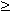Example: Not recognizing intersection graphs of boxes in R2

As for many geometric intersection models, the first step, finding sufficiently many star graphs, is easy for intersection graphs of boxes in Rd. We only have to find the cliques. The problem is the layout step, where we have to place the cliques on the plane in a certain way. After the placement has been done, for every vertex x of G, the (points corresponding to the) cliques containing x generate a smallest axis-parallel rectangle, which we denote by Sx. These rectangles should obey:

• for every vertex x of G, all (points corresponding to) cliques in Sx must contain x, and
• if Sx and Sy intersect, then they have some (point corresponding to a) clique in common.

Actually recognizing intersection graphs of d-dimensional boxes is NP-complete for d => 2 [K94] for d=2, [Y82] for d3). Therefore this layout problem must be NP-complete too.

Erich Prisner